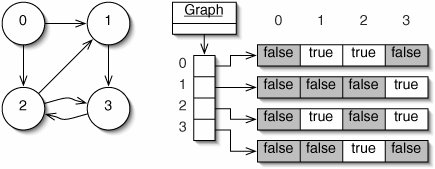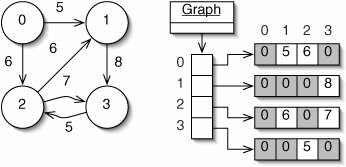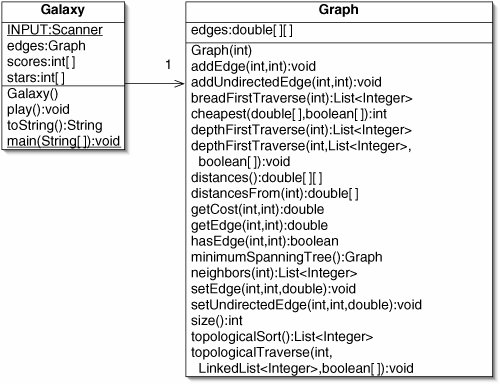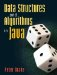# Representation

In representing graphs, we assume that the vertices are numbered 0 through v 1. If it is necessary to associate other data such as city names with the vertices, this can be done with a Map or a direct address table.

In an unweighted graph, we need to keep track only of which vertices are the neighbors of each vertex. The first approach to doing this is the neighbor list representation (Figure 15-8), also known as the adjacency list representation. There is an array containing a chain of ListNodes for each vertex. Each list contains the indices of the neighboring vertices. The amount of memory used is in Q(v + e). The time to check for a particular edge is in O(e).

Figure 15-8. A graph (left) and its neighbor list representation (right).If a graph is dense, it is better to use a neighbor matrix (Figure 15-9), also known as an adjacency matrix. This is essentially a two-dimensional array of booleans. Element <i, j> of this array is true when there is an edge from vertex i to vertex j. The memory used by this representation is in Q(n2), which is more than the neighbor list representation if the graph is sparse. On the other hand, the time to check for a particular edge is constant.

Figure 15-9. A graph (left) and its neighbor matrix representation (right).

(This item is displayed on page 413 in the print version)An added advantage of the neighbor matrix representation is that it is easily adapted to handle weighted graphs (Figure 15-10). Instead of storing a boolean at each position, we store the weight of the corresponding edge, or 0 if there is no edge.

Figure 15-10. A weighted graph (left) and its neighbor matrix representation (right).Code for the neighbor matrix representation is given in Figure 15-11. It can be used to represent an unweighted graph (with the methods addEdge() and hasEdge()) or a weighted graph (with the methods setEdge() and getEdge()). The methods addUndirectedEdge() and setUndirectedEdge() allow us to represent undirected edges as well.

Figure 15-11. Beginning the Graph class.

(This item is displayed on pages 414 - 415 in the print version)

 ``` 1 /** A potentially weighted graph. */ 2 public class Graph { 3 4 /** 5 * edges[i][j] is the weight of the edge from i to j, or 0 if 6 * there is no such edge. 7 */ 8 private double[][] edges; 9 10 /** The argument is the number of vertices in this Graph. */ 11 public Graph(int vertices) { 12 edges = new double[vertices][vertices]; // All zero by default 13 } 14 15 /** Add an edge of weight 1 from i to j. */ 16 public void addEdge(int i, int j) { 17 edges[i][j] = 1; 18 } 19 ``` 20 /** Add edges of weight 1 from i to j and from j to i. */ 21 public void addUndirectedEdge(int i, int j) { 22 edges[i][j] = 1; 23 edges[j][i] = 1; 24 } 25 26 /** Return the weight of the edge from i to j. */ 27 public double getEdge(int i, int j) { 28 return edges[i][j]; 29 } 30 31 /** Return true if there is an edge from i to j. */ 32 public boolean hasEdge(int i, int j) { 33 return edges[i][j] != 0.0; 34 } 35 36 /** Set the weight of the edge from i to j. */ 37 public void setEdge(int i, int j, double weight) { 38 edges[i][j] = weight; 39 } 40 41 /** Set the weight of the edge from i to j and from j to i. */ 42 public void setUndirectedEdge(int i, int j, double weight) { 43 edges[i][j] = weight; 44 edges[j][i] = weight; 45 } 46 47 /** Return the number of vertices in this Graph. */ 48 public int size() { 49 return edges.length; 50 } 51 52 }

It will often prove useful to obtain a List of the neighbors of a vertex, so we provide a method for this (Figure 15-12).

We will add quite a few additional methods to this class, but we have enough now to write the Galaxy program. A UML class diagram is given in Figure 15-13.

The fields and main() method for the Galaxy class are given in Figure 15-14.

The constructor (Figure 15-15) is rather lengthy because it has to specify all of the edges on the board. Line 3 creates a Graph with 20 vertices but no edges. Lines 424 add the edges. Specifically, a two-dimensional array of ints is created and the rows are traversed using an enhanced for loop. Each row contains two numbers, which are passed to addUndirectedEdge() on line 23. On line 25, scores has length 3 so that we can refer to scores and scores; scores is not used. Line 26 allocates the array stars. Line 27 gives a random star to player 1. The loop on lines 2834 gives a random star to player 2; it usually runs only once, but it is set up to try again if player 2 happens to get the same star as player 1.

Figure 15-12. The neighbors() method.

 ``` 1 /** Return a List of the neighbors of vertex i. */ 2 public List neighbors(int i) { 3 List result = new ArrayList(); 4 for (int j = 0; j < size(); j++) { 5 if (hasEdge(i, j)) { 6 result.add(j); 7 } 8 } 9 return result; 10 } ```

Figure 15-13. UML class diagram showing the Galaxy and Graph classes. For completeness, all of the Graph methods are shown, even those not used in Galaxy.Figure 15-14. Fields and main() method for the Galaxy class.

 ``` 1 import java.util.Scanner; 2 3 /** The game of Galaxy. */ 4 public class Galaxy { 5 6 /** For reading from the console. */ 7 public static final Scanner INPUT = new Scanner(System.in); 8 9 /** Edges linking the stars together. */ 10 private Graph edges; 11 12 /** Points earned so far by each player. */ 13 private int[] scores; 14 15 /** Number of player controlling each star, or zero if none. */ 16 private int[] stars; 17 18 /** Create and play the game. */ 19 public static void main(String[] args) { 20 Galaxy game = new Galaxy(); 21 System.out.println("Welcome to Galaxy. "); 22 game.play(); 23 } 24 25 } ```

Figure 15-15. Constructor for the Galaxy class.

 ``` 1 /** Build the galaxy and give one star to each player. */ 2 public Galaxy() { 3 edges = new Graph(20); 4 for (int[] pair : new int[][] 5 {{0, 16}, {0, 17}, {0, 19}, 6 {1, 2}, {1, 8}, 7 {2, 4}, {2, 5}, {2, 8}, 8 {3, 8}, {3, 9}, {3, 16}, 9 {4, 5}, {4, 8}, 10 {5, 6}, {5, 7}, 11 {6, 7}, 12 {7, 8}, {7, 10}, {7, 11}, {7, 12}, {7, 18}, 13 {8, 10}, {8, 14}, 14 {9, 10}, {9, 13}, 15 {10, 12}, {10, 13}, 16 {11, 14}, 17 {11, 19}, 18 {12, 14}, {12, 16}, {12, 19}, ``` 19 {13, 15}, {13, 16}, 20 {15, 16}, {15, 18}, 21 {16, 17}, 22 {18, 19}}) { 23 edges.addUndirectedEdge(pair, pair); 24 } 25 scores = new int; // Initially all zeroes 26 stars = new int; // Initially all zeroes 27 stars[(int)(Math.random() * 20)] = 1; 28 do { 29 int star = (int)(Math.random() * 20); 30 if (stars[star] == 0) { 31 stars[star] = 2; 32 return; 33 } 34 } while (true); 35 }

In writing the toString() method (Figure 15-16), we opt for a simple table.

Figure 15-16. The toString() method from the Galaxy class.

 ``` 1 public String toString() { 2 StringBuilder result = new StringBuilder(); 3 result.append("Star Owner Neighbors "); 4 for (int i = 0; i < 20; i++) { 5 result.append(i + " " + stars[i] + " " 6 + edges.neighbors(i) + " "); 7 } 8 for (int p = 1; p <= 2; p++) { 9 result.append("Player " + p + ": " + scores[p] + " "); 10 } 11 return result.toString(); 12 } ```

The play() method (Figure 15-17) is one of the simpler ones we've written. In the loop, lines 68 read a star number from the user. Line 9 gives the user control of that star. Lines 1014 add any points scored. Line 15 toggles whose turn it is.

Exercises

 15.9 How can the neighbor list representation (Figure 15-8) be modified to handle weighted graphs?

Figure 15-17. The play() method from the Galaxy class.

 ``` 1 /** Play the game. */ 2 public void play() { 3 int player = 1; 4 for (int turn = 0; turn < 18; turn++) { 5 System.out.println(this); 6 System.out.print("Player " + player + ", pick a star: "); 7 int star = INPUT.nextInt(); 8 INPUT.nextLine(); // To clear out input 9 stars[star] = player; 10 for (int s : edges.neighbors(star)) { 11 if (stars[s] == 3 - player) { 12 scores[player]++; 13 } 14 } 15 player = 3 - player; 16 } 17 System.out.println(this); 18 } ```
 15.1 What is the order of the running time of the neighbors() method (Figure 15-12)? 15.11 A graph could be represented as a List of edges, with each edge containing the indices of the two vertices connected by the edge. How much memory is used by this representation? How long does it take to check for the existence of a particular edge? How can this representation be modified to handle weighted graphs?Data Structures and Algorithms in Java
ISBN: 0131469142
EAN: 2147483647
Year: 2004
Pages: 216
Authors: Peter Drake